# Friction - JEE Main Previous Year Questions with Solutions

JEE Main Previous Year Question of Physics with Solutions are available here. Practicing JEE Main Previous Year Papers Questions of Physics will help all the JEE aspirants in realizing the question pattern as well as help in analyzing their weak & strong areas. Get detailed Class 11th &12th Physics Notes to prepare for Boards as well as competitive exams like IIT JEE, NEET etc. eSaral helps the students in clearing and understanding each topic in a better way. eSaral is providing complete chapter-wise notes of Class 11th and 12th both for all subjects. Besides this, eSaral also offers NCERT Solutions, Previous year questions for JEE Main and Advance, Practice questions, Test Series for JEE Main, JEE Advanced and NEET, Important questions of Physics, Chemistry, Math, and Biology and many more. Download eSaral app for free study material and video tutorials.
Q. A horizontal force of 10 N is necessary to just hold a block stationary against a wall. The coefficient of friction between the block and the wall is 0.2. The weight of the block is-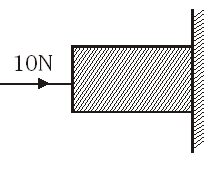(1) 20 N (2) 50 N (3) 100 N (4) 2 N [AIEEE - 2003]
Ans. (4)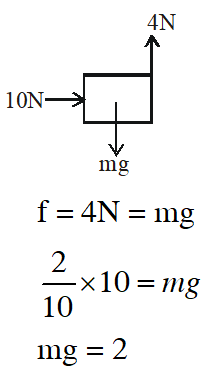Q. A marble block of mass 2 kg lying on ice when given a velocity of 6 m/s is stopped by friction in 10s. Then the coefficient of friction is- (1) 0.02        (2) 0.03       (3) 0.06       (4) 0.01 [AIEEE - 2003]
Ans. (3)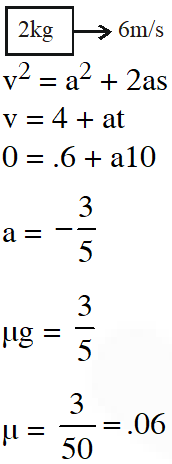Q. A block rests on a rough inclined plane making an angle of $30^{\circ}$ with the horizontal. The coefficient of static friction between the block and the plane is 0.8. If the frictional force on the block is 10 N, the mass of the block (in kg) is : (taken $\left.\mathrm{g}=10 \mathrm{m} / \mathrm{s}^{2}\right)$ (1) 2.0          (2) 4.0           (3) 1.6           (4) 2.5 [AIEEE - 2004]
Ans. (1)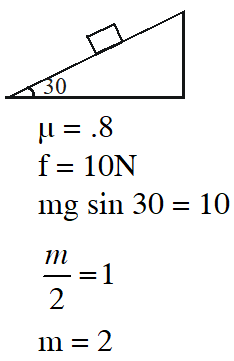Q. A smooth block is released at rest on a $45^{\circ}$ incline and then slides a distance d. The time taken to slide is n times as much to slide on rough incline than on a smooth incline. The coefficient of friction is- $(1) \mu_{\mathrm{k}}=1-\frac{1}{\mathrm{n}^{2}}$ $(2) \mu_{\mathrm{k}}=\sqrt{1-\frac{1}{\mathrm{n}^{2}}}$ (3) $\mu_{\mathrm{s}}=1-\frac{1}{\mathrm{n}^{2}}$ (4) $\mu_{\mathrm{s}}=\sqrt{1-\frac{1}{\mathrm{n}^{2}}}$ [AIEEE - 2005]
Ans. (1)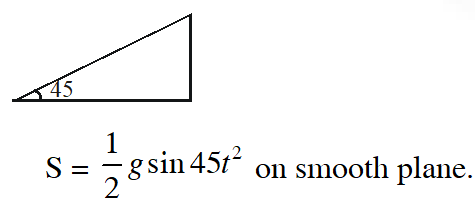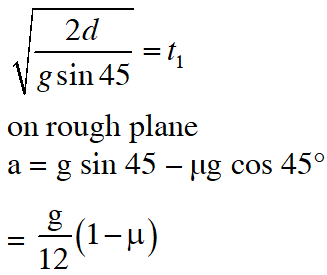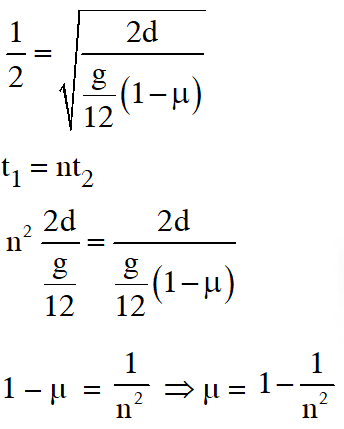Q. The upper half of an inclined plane with inclination $\phi$ is perfectly smooth, while the lower half is rough. A body starting from rest at the top will again come to rest at the bottom, if the coefficient of friction for the lower half is given by- (1) $2 \sin \phi$ (2) $2 \cos \phi$ (3) 2 tan $\phi$ (4) $\tan \phi$ [AIEEE - 2005]
Ans. (3)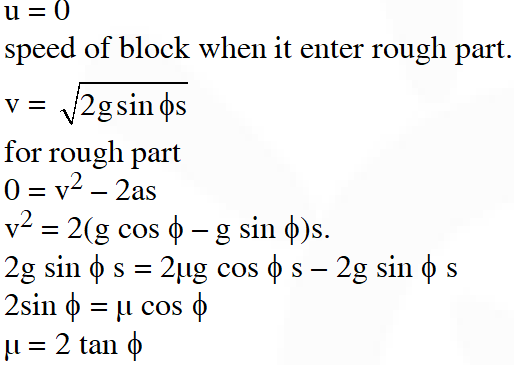Q. Consider a car moving on a straight road with a speed of 100 m/s. The distance at which car can be stopped, is : $\left[\mu_{\mathrm{k}}=0.5\right]$ (1) 800 m          (2) 1000 m         (3) 100 m           (4) 400 m [AIEEE - 2005]
Ans. (2)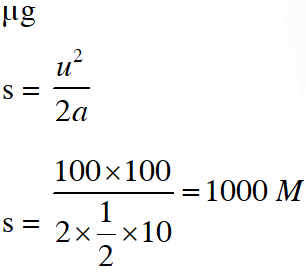Q. The minimum force required to start pushing a body up a rough (frictional coefficient $\mu$) inclined plane is $\mathrm{F}_{1}$ while the minimum force needed to prevent it from sliding down is $\mathrm{F}_{2}$. If the inclined plane makes an angle $\theta$ from the horizontal such that $\tan \theta$ = $2 \mu$then the ratio $\frac{\mathrm{F}_{1}}{\mathrm{F}_{2}}$ is :- (1) 4 (2) 1 (3) 2 (4) 3 [AIEEE - 2011]
Ans. (4)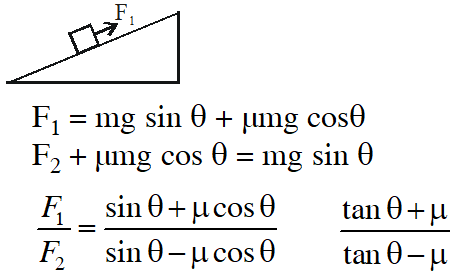$=\frac{2 \mu+\mu}{2 \mu-\mu}=\frac{3 \mu}{\mu}=3$
Q. A block of mass m is placed on a surface with a vertical cross section given by $\mathrm{y}=\frac{\mathrm{x}^{3}}{6}$ . If the coefficient of friction is 0.5, the maximum height above the ground at which the block can be placed without slipping is :- (1) $\frac{1}{3} \mathrm{m}$ (2) $\frac{1}{2} \mathrm{m}$ (3) $\frac{1}{6} \mathrm{m}$ (4) $\frac{2}{3} \mathrm{m}$ [jee-main-2014]
Ans. (3)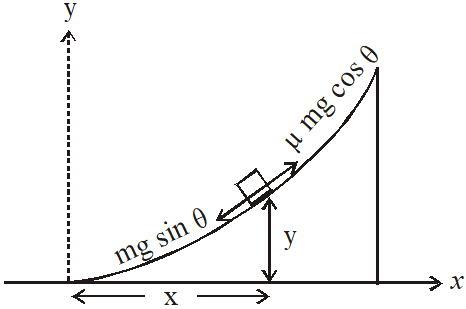For equilibrium under limiting friction $\operatorname{mg} \sin \theta=\mu \operatorname{mg} \cos \theta$ $\Rightarrow \tan \theta=\mu$ From the equation of surface $y=\frac{x^{3}}{6}$ slope $=\frac{d y}{d x}=\frac{3 x^{2}}{6}=\tan \theta$ $\Rightarrow \frac{\mathrm{x}^{2}}{2}=\mu=0.5 \Rightarrow \mathrm{x}=1$ So $\mathrm{y}=\frac{1}{6}$
Q. Given in the figure are two blocks A and B of weight 20 N and 100 N, respectively. These are being pressed against a wall by a force F as shown. If the coefficient of friction between the blocks is 0.1 and between block B and the wall is 0.15, the frictional force applied by the wall on block B is :- (1) 120 N (2) 150 N (3) 100 N (4) 80 N [jee-main-2015]
Ans. (1)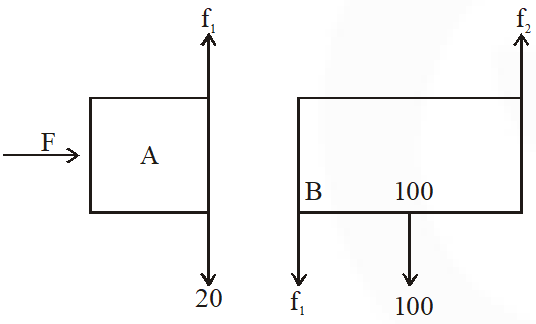for equllibrrium of A $\mathrm{f}_{1}=20$ for equllibrrium of B $\mathrm{f}_{2}=\mathrm{f}_{1}+100$ $\mathrm{f}_{2}=120 \mathrm{N}$
Q. Two masses $\mathrm{m}_{1}$ = 5kg and $\mathrm{m}_{2}$ = 10kg, connected by an inextensible string over a frictionless pulley, are moving as shown in the figure. The coefficient of friction of horizontal surface is 0.15. The minimum weight m that should be put on top of $\mathrm{m}_{2}$ to stop the motion is :-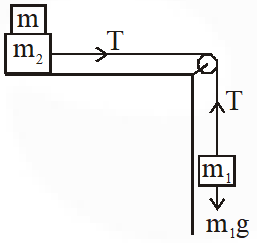(1) 27.3 kg (2) 43.3 kg (3) 10.3 kg (4) 18.3 kg [JEE-(Mains) - 2018]
Ans. (1)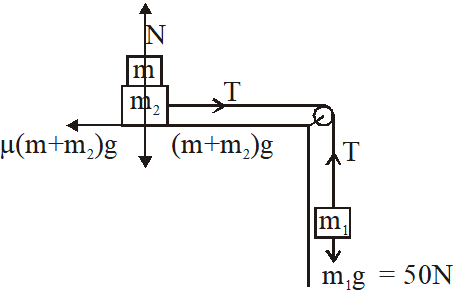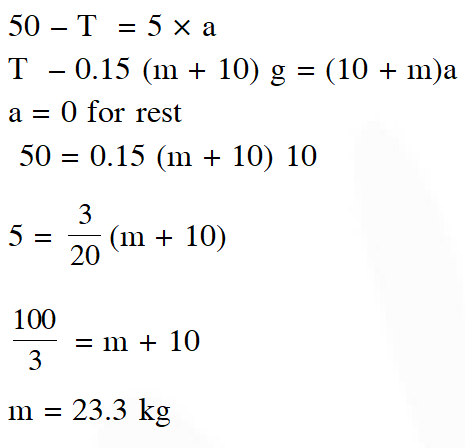Safa
Sept. 24, 2021, 10:31 p.m.
Best for jee aspirants
fuck off esaral
April 29, 2021, 1:40 p.m.
Useless questions just for beginners these are the simplest questions selected from different years these kind of question are not going to come in your jee prep or jee advanced or even mains
bjjugu
Feb. 4, 2021, 1:47 p.m.
uuuuuuuuulu,iug
Himanshi saxena
Jan. 22, 2021, 5:16 a.m.
Great help thanks alot
Priyanshu
Jan. 10, 2021, 1:18 p.m.
Thanxs sir....
Roshni
Jan. 10, 2021, 10:24 a.m.
Nice questions can you give such more questions
Aashika sharma
Dec. 29, 2020, 10:58 p.m.
It is so helpful for a jee aspirant.
Aayush
Dec. 28, 2020, 3:23 p.m.
Very good questions thanks a lot
Sunil
Dec. 20, 2020, 12:15 a.m.
Nice sir
Shreyansh
Dec. 10, 2020, 11:46 a.m.
Good
Prashant
Nov. 10, 2020, 9:14 p.m.
Nice questions
Prashant
Oct. 25, 2020, 1:25 p.m.
Anshul
Oct. 11, 2020, 8:30 p.m.
imemyself
Oct. 10, 2020, 8:11 p.m.
good practice questions !!!
Asha
Sept. 27, 2020, 10:10 p.m.
Could you please keep many more previous year questions topic wise.
me
Sept. 23, 2020, 5:43 p.m.
nikl
CHANDRA SAILESH
Sept. 14, 2020, 7:27 a.m.
Thank you very good questions
SHARATH
Sept. 12, 2020, 11:36 a.m.
EASY QUESTIONS FOR JEE MAINS
Venkanna
Sept. 11, 2020, 7:49 p.m.
Can u plz upload past 2 papers of mains
Nikitha
Sept. 8, 2020, 3:55 p.m.
Good
Xyz
Aug. 14, 2020, 10:58 p.m.
Option in last question is wrong, correct it.
Manas
Aug. 12, 2020, 9:11 a.m.
Graeat work
Sudheer
Aug. 11, 2020, 1:54 p.m.
Good revision
LAXMI PRASANNA ANNEPU
Aug. 9, 2020, 5:54 p.m.
Esther Evangeline
Aug. 7, 2020, 1:47 p.m.
manu
Aug. 5, 2020, 11:01 p.m.
sir,the 4 th qs soln has one error,its g/root 2 and not g/12
ayush
July 22, 2020, 12:53 p.m.
it was really helpful ....i enjoyed a lot
VARUN DUBE
July 12, 2020, 11:06 a.m.
Thanks, IT WAS REALLY HELPFUL..!! HERE, I got the most popular and most frequently seen questions..!! THANKS FOR THE FAQs provided ...!!!!
Bindu
July 11, 2020, 3:40 p.m.
Less question can't able to practice well because still didnt expose to many questions
Anubhav
July 7, 2020, 5:45 a.m.
The answer of the last question is not matching with the options . Solution is right but it is not in the option . please correct it.
Ankur
June 3, 2020, 3:01 p.m.
Best site
Nikki
May 27, 2020, 9:59 a.m.
Good
May 15, 2020, 2:02 p.m.
Good
panakj
May 9, 2020, 5:31 a.m.
good
jappos
May 4, 2020, 1:26 p.m.
v good
sai bharath
April 28, 2020, 5:31 p.m.
good
M. Gowtham
April 15, 2020, 1:04 p.m.
Good
sam
April 3, 2020, 1:33 p.m.
hi